# What are saturation force harmonics?

## Definition

In e-NVH, saturation force harmonics refer for Maxwell force harmonics linked to magnetic saturation combined with slotting effects. Saturation tends to flatten the airgap flux, resulting in a modification of the harmonic content of Maxwell stress. In particular airgap permeance harmonics of wavenumbers 2p appear.

More precisely, as any force harmonic is due to the product of two flux density harmonics B1 and B2, these saturation harmonics can be written as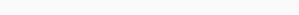where

•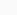is a saturation permeance harmonic
•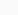is a slotting permeance harmonics
•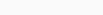is the fundamental magnetomotive force

As the noise and vibration frequencies are the same as the exciting force frequencies, saturation harmonics can also refer to some specific harmonics of the acoustic noise spectrum or vibration velocity spectrum.

Saturation harmonics should disappear when the machine runs in linear conditions (low magnetic excitation).

## Application to e-NVH

In induction machines at no-load, slotting harmonics can be more precisely derived as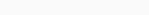where

•stands for saturated permeance harmonic
•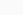stands for combined rotor and stator slot permeance harmonic
•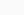is the fundamental stator magnetomotive force

One can show that the resulting force wavenumbers r and frequencies f are given for the first saturated permeance harmonic by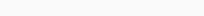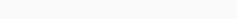where

•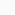(resp.) is the rank of stator (resp. rotor) permeance harmonic (0 for DC permeance, 1 for highest magnitude permeance harmonic)
•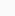is the fundamental electrical frequency
•(resp) is the stator (resp. rotor) slot number
•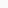is the fundamental slip

Saturation harmonics can therefore interfere with some slotting harmonics.
In spectrograms saturation harmonics appear as straight lines crossing the origin:

## Noise control action

Reducing the saturation levels allows to cancel the magnetic force excitation due to saturation, it can be obtained by a change of the control law, modification of the magnetic circuit geometry or change of magnetic sheet material.
Besides, changing the slot/pole combination has also an influence on these specific excitations as they combine both saturation and slotting effects.

## Application to MANATEE

MANATEE software electromagnetic models can account for saturation effects using non linear finite element calculations or calculating the saturated permeance wave based on semi analytical techniques.